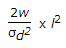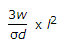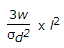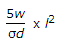# Civil Engineering - Theory of Structures - Discussion

Discussion Forum : Theory of Structures - Section 1 (Q.No. 14)
14.
Keeping the depth d constant, the width of a cantilever of length l of uniform strength loaded with a uniformly distributed load w varies from zero at the free end andat the fixed endat the fixed endat the fixed endat the fixed end
Explanation:
No answer description is available. Let's discuss.
Discussion:
21 comments Page 1 of 3.

Abiy said:   9 months ago

Abiy said:   9 months ago
@Gupta.

Thanks for your explanation but for load w uniformly distributed from free end zero.
W=wl/2.
Which it's Mmax=wl/3 At the fixed end.
(1)

Jeganathan said:   2 years ago
Sigma is nothing but f (bending stress).
And Z(section modulus) = bd2/6.
Then,

B.M. at fixed support is wl2/2.
M= f.(I/Y)
M=f.Z
wl2/2 = f * Z.
wl2/2 = f * bd2/6.
b= (3wl2)/fd2.

(3)

Bishwo said:   2 years ago
Bending moment at fixed =1/3 (wl^2) /2.

1/3 is because it's a triangular slab of thickness d and with b. 1 part of whole rectangle will give BM at the fixed end.
(1)

Michael said:   2 years ago
Thanks @Anshul Gupta.

SHUBHAM SIDDHARTH NAYAK said:   3 years ago
Cantilever carrying UDL- BM at fixed end = wl^2 /2.
We know, M = fz.
Wl^2/2= f *(bd^2/6),
b =3wl^2/fd^2.

Syed Mazhar said:   3 years ago
@Dhananjay.
From bending eq
M/I= f/y=E/R
M=(I/y)f
M=zf.

Dhananjay said:   4 years ago
@Anshul,

How can you equate BM with S*Z? Here, units are different. Please explain.

Nilraj. said:   5 years ago
@Ravi.

Narayan said:   5 years ago
s= σ(direct stress).

M= wl^2-1/2*(l*w)*2/3(l)
= wl^2/6.

But,
M=S * Z.
wl^2/6 = S * b * d^2/6.
b = wl^2/s * d^2.